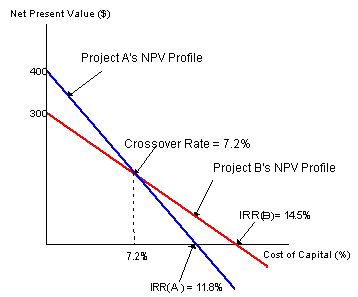### Why should I choose AnalystNotes?

AnalystNotes specializes in helping candidates pass. Period.

##### Subject 5. Comparison of the NPV and IRR Methods
The IRR formula is simply the NPV formula solved for the particular rate that sets the NPV to 0. The same equation is used for both methods.

The NPV method assumes that cash flows will be reinvested at the firm's cost of capital, while the IRR method assumes reinvestment at the project's IRR. Reinvestment at the cost of capital is a better assumption in that it is closer to reality.For independent projects, the NPV and IRR methods indicate the same accept or reject decisions. Assuming that Project A and B are independent, consider their NPV profiles.

• The IRR criterion for accepting an independent project is IRR > hurdle rate. That is, the cost of capital must be less than (or to the left of) the IRR.
• Whenever the cost of capital is less than the IRR, the project's NPV is positive. Recall the decision rule for independent projects: accept if NPV > 0. Thus, both projects should be accepted based on the NPV method.

However, for mutually exclusive projects, ranking conflicts can arise. Assuming that Project A and B are mutually exclusive, consider their NPV profiles.

• If the cost of capital > crossover rate, then NPVB > NPVA and IRRB > IRRA. Thus, both methods lead to the selection of Project B.
• If the cost of capital < crossover rate, then NPVB < NPVA and IRRB > IRRA. Thus, a conflict arises because now the NPV method will select Project A while the IRR method will choose B.
• Therefore, for mutually exclusive projects, the NPV and IRR methods lead to same decisions if the cost of capital > the crossover rate and different decisions if the cost of capital < the crossover rate.

For mutually exclusive projects, the NPV and MIRR methods will lead to the same accept or reject decision when:

• Two projects are of equal size and have the same life.
• Two projects are of equal size but differ in lives.

However, the projects can generate conflicting results if the NPV profiles of two projects cross (and there is a crossover rate):

• As long as the cost of capital (k) is larger than the crossover rate, the two methods both lead to the same decision;
• A conflict exists if k is less than the crossover rate.

Two conditions cause the NPV profiles to cross:

• When project size (or scale) differences exist. The cost of one project is larger than that of the other.
• When timing differences exist. The timing of cash flows from the two projects differs in that most of the cash flows from one project come in the early years while most of the cash flows from the other project come in the later years.

The root cause of the conflict between NPV and IRR is the rate of return at which differential cash flows can be reinvested. Both the NPV and IRR methods assume that the firm will reinvest all early cash flows. The NPV method implicitly assumes that early cash flows can be reinvested at the cost of capital. The IRR method assumes that the firm can reinvest at the IRR.

Whenever a conflict exists, the NPV method should be used. It can be demonstrated that the better assumption is the cost of capital for the reinvestment rate (Hint: don't focus too much on this topic, as it is beyond the scope of the CFA exam).

Multiple IRRs is a situation where a project has two or more IRRs. This problem is caused by the non-conventional cash flows of a project.

• Conventional cash flows means that the initial cash outflows are followed by a series of cash inflows.
• Non-conventional cash flows means that a project calls for a larger cash outflow either sometime during or at the end of its life. Thus, the signs of the net cash flows flip-flop during the project's life.

In fact, non-conventional cash flows can cause other problems, such as negative IRR or an IRR that leads to an incorrect accept or reject decision. However, a project can have only one NPV, regardless of its cash flow patterns, so the NPV method is preferable when evaluating projects with non-normal cash flows.

Learning Outcome Statements

e. explain the NPV profile, compare the NPV and IRR methods when evaluating independent and mutually exclusive projects, and describe the problems associated with each of the evaluation methods;

CFA® 2022 Level II Curriculum, , Volume 4, Reading 32